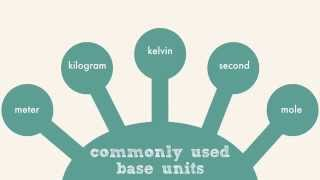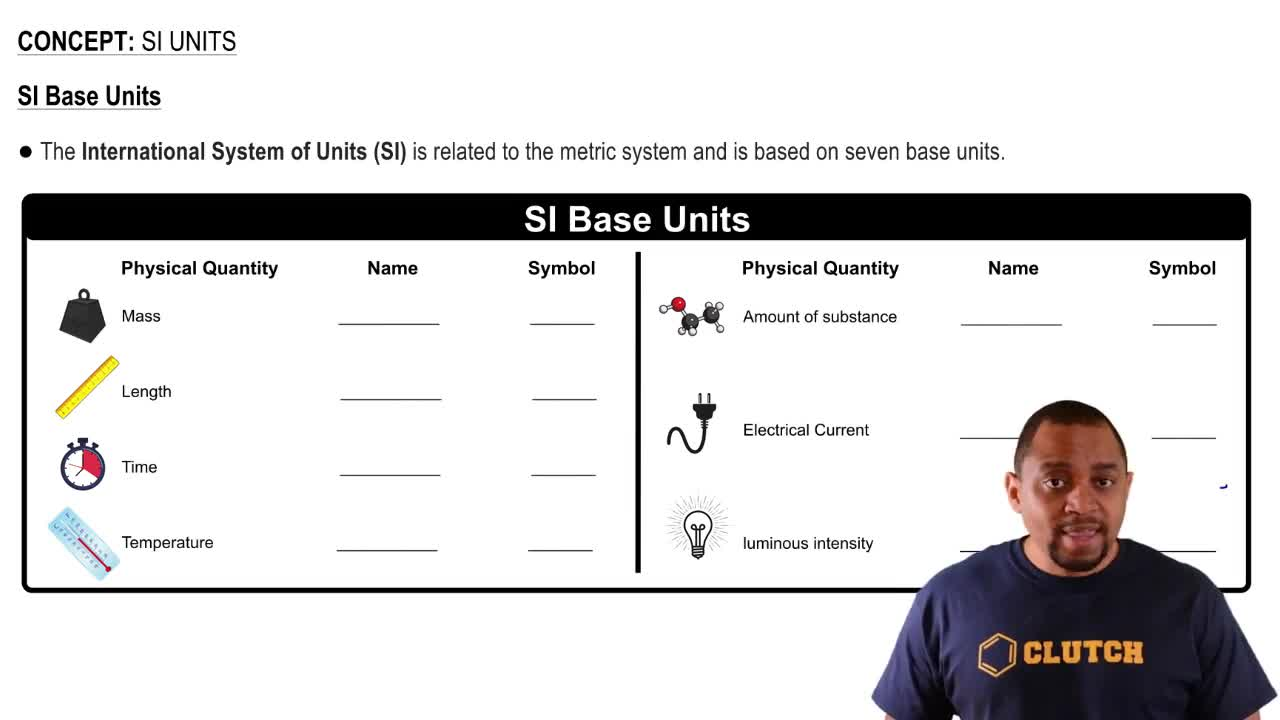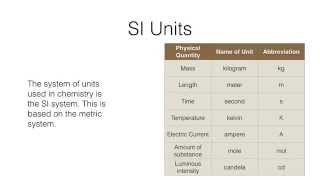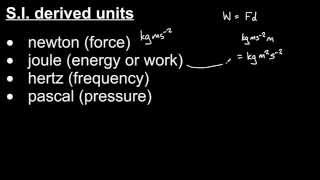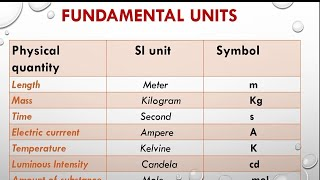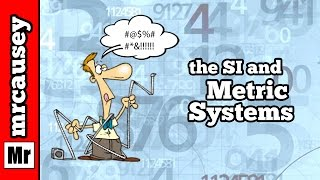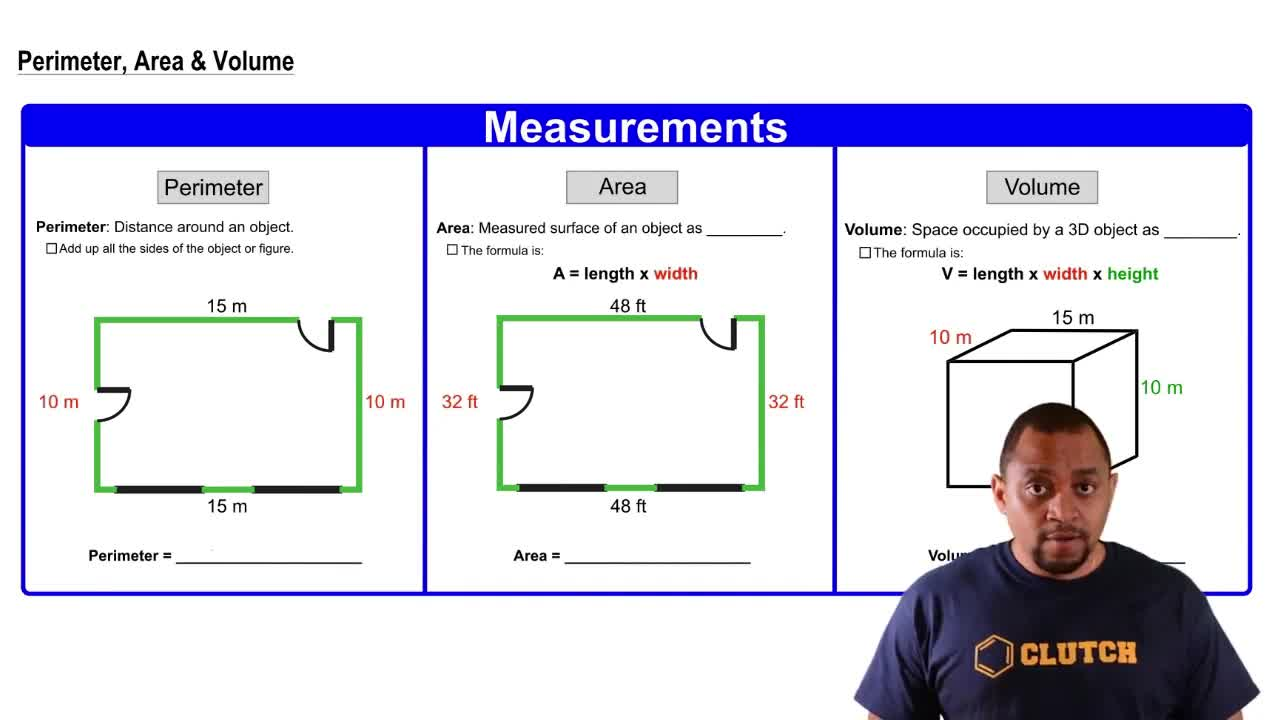Start typing, then use the up and down arrows to select an option from the list.
1. 1. Intro to General Chemistry2. SI Units
Problem

# What is the difference between a derived SI unit and a funda-mental SI unit? Give an example of each

Relevant Solution48s
Play a video:
Hello everyone today. We have the following question which of the following statements is true about derived S. I. Unit and fundamental S. I. Unit. So the answer here is going to be be a derived S. I. Unit is a combination of two or more based based S. I. Units, for example speed, which measured in meters per second, while there are only seven fundamental S. I. Units, for example length in meters. So once again a derived S. I. Unit is going to be a combination of based units while the fundamental S. I. Units are going to be the more simple terms, one as high units such as length, which is measured in meters. And with that we've answered the question. I hope this helped and until next time.